# Effects of the Geometry Scale on the Behaviour of the Local Physical Process of the Velocity Field in the Laminar Flow

Effects of the Geometry Scale on the Behaviour of the Local Physical Process of the Velocity Field in the Laminar Flow

Yahia Lasbet Lakhdar Aidaoui Khaled Loubar

Laboratoire de Développement en Mécanique et Matériaux (LDMM), Université de Djelfa 17000, Algeria

Ecole des Mines de Nantes, 44307 Nantes Cedex 3, France

Corresponding Author Email:
peeryahia@yahoo.fr
Page:
439-445
|
DOI:
https://doi.org/10.18280/ijht.340313
|
Accepted:
|
Published:
30 September 2016
| Citation

OPEN ACCESS

Abstract:

It is well known that the convective terms in the equation of fluid motion play an outstanding role on the local proprieties of flows and they affect the local behaviour of physical processes such as deformation, rotation, stretching and folding. Large values of these processes in the flow have pronounced and lasting effects on the improvement of heat transfer and fluid mixing in ducts. The modification of the geometric scale presents an easy and adequate solution to increase these parameters. For this purpose, three dimensional zigzag channels with hydraulic diameters equal to 5 mm, 10 mm and 20 mm were examined in this study. Evolutions of the deformation rate, rotation rate and the stretching/compression coefficients of the vortices were examined for different values of the Reynolds number in three dimensional laminar open flow, using a CFD code. The results illustrate that the geometry with the smallest hydraulic diameter is the more favourable to increase the considered parameters.

Keywords:

Deformation, Rotation, Stretching/Compression, Complex geometry, Chaotic advection, Pressure losses.

1. Introduction

One broadly applicable and mostly reputed way to improve heat and mass transfer processes in fluids is to create the Lagrangian chaos in flows called chaotic advection or sub-laminar flow    . This technique does not require the insertion of static mixers contrary to the conventional way .  Aref  outlined the chaotic kinematic of fluid particle trajectories in the two-dimensional periodic flows using Poincaré section as one of the important tools for the analysis of dynamic systems . After this work, many researches have been carried out to highlight the local proprieties of the velocity field in such flow and its effects on the enhancement of fluids mixing and heat transfer improvement. For example, in , a study of two-dimensional problem of fluid diffusion into another fluid was performed. The results reported that an intense deformation and rotation rates lead to a good mixing of the two fluids. Toussaint et al  studied the unsteady three-dimensional flow in a rectangular cavity where the authors reported the evolution of the energy spectrum with the wave number. They found that the chaotic advection creates many scales in the flow and transferring energy from a given scale to the scale smaller. Besides, the dissipated energy in this type of flows is very low compared to that created in turbulent flows, even if the fluid is very viscous . It is found that the chaotic advection enhances considerably the agitation and the stirring in the steady laminar incompressible flow case, within a twisted pipe with circular cross-section . It was demonstrated that this enhancement is realized without any external intervention or additive energy expenditure, contrary to the turbulent flows case.

Habchi et al  studied the mixing between a dispersed phase (water) and a continuous phase (oil) in helically coiled and chaotic twisted pipes. Deformation rate and elongation were estimated to verify the breakup of the droplets. Also, they checked through Eulerian and Lagrangian analytical approaches, that chaotic advection drives fluid particles to visit regions of high shear and elongation rates. Castelain et al  performed an experimental study of laminar flow in twisted pipe with a local Eulerian approach. The obtained results revealed that the fluid flow creates a complex stretching and folding phenomena, which produces horseshoe-type maps (e.g. two very close particles diverge exponentially).

Habchi et al  studied numerically two types of channels. The first one is constituted by several arrays of rectangular vortex generators which are mounted in-line. In the second one, the arrays are periodically rotated by an angle of 90, with respect to the pipe axis. In this work, the authors have calculated the Poincaré maps, helicity (H) and the vortex intensity $(\Omega)$ , in both geometries. They found that the fluid flow in the second geometry is chaotic and leads to a good mixing in its cross section.

Lasbet et al [14-15] presented the evolution of the trajectory of two particles, which were initially close to each other and injected at the inlet of a C-shaped geometry. The geometry cross section was rectangular with an aspect ratio of 2 and a hydraulic diameter of 1.33mm.  In this work, the particles trajectories were diverging rapidly; this can be a clear sign of chaotic advection as reported by . The chaotic behaviour of fluid trajectories in the C-shaped geometry produces the greatest heat transfer intensification (the convective heat transfer coefficient was six times higher compared to that found in the straight channel).

In order to optimize the geometry previously studied by [4-14-15], the present numerical work is carried out to analyse the behaviour of the velocity field and its proprieties. More specifically, the current work focuses on the effect of the geometry scale on the evolutions of the flow local kinematic, presented in terms of rotation rate, deformation rate and stretching/folding.

2. Description of The Geometry and Numerical Method

In order to examine the scale geometry effect on the flow structure, three geometries having the same pattern with different dimensions are considered in this study: C05, C10 and C20 corresponding to a hydraulic diameter of 5mm, 10mm and 20 mm respectively. Each geometry is characterised by a square cross-section with an aspect ratio equal to 1 (Figure 1). The selected geometry is formed by two geometrical perturbations and each one is composed of a succession of three straight bends. The exit of the first perturbation is at the plane-4 while the exit of the second perturbation is at the plane-8. Because of the successive sudden reorientation of the flow direction in each disturbance, the baker's transformation in the fluid flow can be predicted. Such transformations highlight the stretching and folding phenomena where the trajectories of fluid particles become chaotic as proven by .

## figure_1.png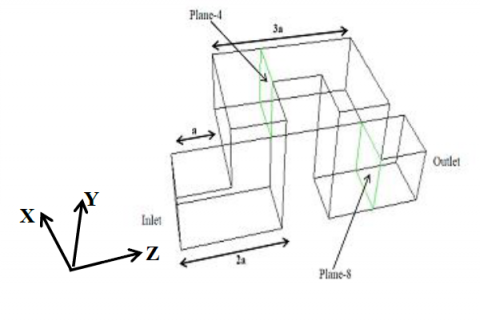Figure 1. The considered geometry, C-shape form

The mass conservation and Navier–Stokes equations were numerically solved using the commercial CFD code Fluent© and are given by the following equations respectively:

$\vec{\nabla} \cdot \vec{V}=0$(1)

$\vec{V} \cdot \bar{\bar{\nabla}}\vec{V}=-\frac{1}{ρ}\vec{\nabla} P+v\Delta\vec{V}$ (2)

The applied boundary conditions are as follow:In the present study, the fluid is considered as Newtonian and incompressible and the fluid flow regime is laminar and steady.

• At the inlet section: fully developed velocity profile .
• At solid walls: no–slip conditions.
• At the outlet section: outflow condition is considered.

In our previous study , we characterized this geometry experimentally and numerically, in terms of heat transfer. Numerical and experimental Results are in good agreement and they show best thermal performances compared to other geometries considered in .

A previous sensitivity mesh study performed by  shown that for each cubic cell with dimensions (a,a,a) corresponding to the three directions (x,y,z), the spatial resolution (40.40.40) is the appropriate grid to detect the velocity and temperature gradients in the fluid flow.

The SIMPLE scheme is used to achieve the pressure-velocity coupling, while in the spatial discretization, a Second-Order upwind scheme is adopted for momentum and pressure.

During the numerical simulations, it is considered that the convergence is achieved when the residues are less than 10-6 for the conservation equations.

3. Results and Discussions

3.1 Stretching and compression of the vorticity

Applying the curl operation on the Navier-Stokes equation leads to the vortex transfer equation, which can be written in the following form:

$∂\vec{Ω}/∂t+\vec{V}.\bar{\bar{∇}}\vec{Ω}=\vec{Ω}.\bar{\bar{∇}}\vec{V}+νΔ\vec{Ω}$(3)

The term $\vec{Ω}.\bar{\bar{∇}}\vec{V}$ induces formation of vortex structures in the flow with different sizes by generating the stretching and compression (folding) vortex in the flow, see figure 2. The stretching and compression phenomena act simultaneously on the vortex dimensions. At a given time, the stretching operation, increases the vortex length and decreases its cross section, while the compression decreases the vortex length and increases its cross section. The diminution of the cross-section engenders the vortex intensity augmentation. These phenomena are generated as a consequence of the conservations of the mass and angular momentum. As a result, a large cascade of scale in the flow is produced, where the large scale size corresponds to the production scale, which is limited by the flow domain size. The smaller scale is related to the dissipation scale and it is limited by the viscous effects. The growing influence of the convective terms in equation (3) leads to the transition regime from laminar to turbulence.

## figure_2.pngFigure 2. Illustration of the stretching and compression operations

The appearance of the stretching and folding in the flow often gives rise to chaotic behaviour. Stretching results in nearby points diverging, folding results in distant points being mixed together. These operations in the flow destroy the thermal and dynamic boundary layers and prevent its re-formation. The boundary layer being a barrier against the parietal thermal transfer, its destruction enhances the heat transfer . On the other hand, these operations increase the contact area between fluids to be mixed even in the existence of the interfacial barrier as surface tension . To characterize this behaviour in the flow, the stretching and compression coefficients of the vortex  $\alpha$  was estimated. It is defined by the following expression:

$\alpha=\frac{\vec{\Omega} \cdot \overline{\overline{D}} \cdot \vec{\Omega}}{\Omega^{2}}$(4)

With $\overline{\overline{D}}$  is the deformation tensor and $\vec{\Omega}$  is the vorticity vector. At any location where $\alpha>0$ , the vortex stretching prevails on vortex compression . $ \cdot \alpha^{+}$  presents the arithmetic average of the positive values of the stretching coefficient and $\alpha^{-}$  presents the arithmetic average of the negative values of the folding coefficient.

Figures 3 and 4, present respectively the evolutions of vortex stretching coefficient $\left(\alpha^{+}\right)$  and compression coefficient $\left(\alpha^{-}\right)$  with respect to Reynolds number (Re) in the three geometries types. These coefficients increase constantly with the increase of the Reynolds number. For the C10 and C20 geometries, the stretching and compression coefficients are barely different mainly at low Reynolds number. Once the Reynolds number exceeds the value of 50, these factors in the C05 channel overcome on that in the other geometries. The decrease of the scale makes these two phenomena very important in the flow where the stretching aids to create grand structures (large scale) and folding aids to create small dissipation structures (small scale). This allows transferring the energy from the large scale to the small scale.

## figure_3.png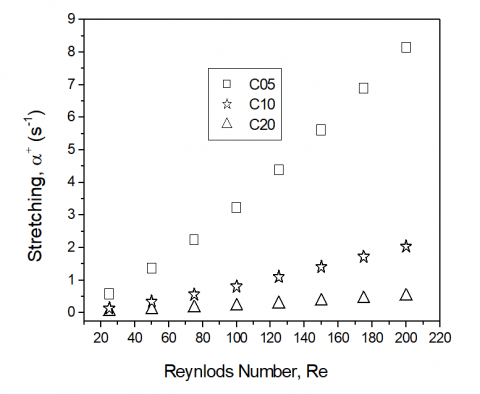Figure 3. Evolution of the vortex stretching in the fluid flow with Reynolds number for the three geometries

## figure_4.png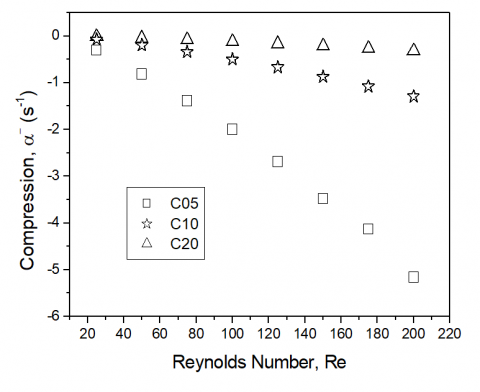Figure 4. Evolution of the vortex compression in the fluid flow with Reynolds number for the three geometries

3.2 Deformation and rotation rates in the flow

Both mechanisms (deformation and rotation) are complementary in the mixing operations. The rotation process gives rise to three-dimensional movements of the fluid particles and it can transport the fluid particles to regions of high shear rates. The rotation process realizes a good macroscopic mixing by agitation while the deformation process achieves a good quality of mixing by molecular diffusion. For this aim, the geometry scale can be a potential solution for increasing the deformation and rotation rates at once. In the present study, an examination of the geometry dimensions effect on the deformation and rotation rates is performed. Evolutions of the mean deformation and rotation rates ( $\left(D_{\mathrm{m}} \text { and } \Omega_{\mathrm{m}}\right)$  in the three geometries (C05, C10 and C20) in function of the Reynolds number ranging from 25 to 200 are presented in figure 5 and 6. The two parameters, rotation and deformation, are defined by  by the following equations:

$D=\left[2\left(\frac{\partial u}{\partial x}\right)^{2}+2\left(\frac{\partial v}{\partial y}\right)^{2}+2\left(\frac{\partial w}{\partial z}\right)^{2}+\left(\frac{\partial u}{\partial y}+\frac{\partial v}{\partial x}\right)^{2}+\left(\frac{\partial u}{\partial z}+\frac{\partial w}{\partial x}\right)^{2}+\left(\frac{\partial v}{\partial z}+\frac{\partial w}{\partial y}\right)^{2}\right]^{\frac{1}{2}}$(5)

$D_{\mathrm{m}}=\frac{1}{\mho} \int D d \mathfrak{\mho}$(6)

$\Omega=\frac{1}{2}\left[\left(\frac{\partial w}{\partial y}-\frac{\partial v}{\partial z}\right)^{2}+\left(\frac{\partial u}{\partial z}-\frac{\partial w}{\partial x}\right)^{2}+\left(\frac{\partial v}{\partial x}-\frac{\partial u}{\partial y}\right)^{2}\right]^{\frac{1}{2}}$(7)

$\Omega_{\mathrm{m}}=\frac{1}{\mho} \int \Omega d \mho$(8)

where $\mho$ represents the total volume of the fluid in the channel.

For the three channels, both quantities evolve with Reynolds number in different manners according to the geometry in which they are regarded. When the Reynolds number increases, these parameters are more vigorous, and the flow becomes more agitated and sheared. Besides, the flow in the channel C05 exhibits very high rates of deformation and rotation compared to the other two ones: C10 and C20. In other words, at a given Reynolds number value, the deformation and rotation rates in the C05 geometry are more important compared to the other geometries. This explains that this behaviour is kinematic and is accentuated by the Reynolds number. The C10 and C20 channels have qualitatively the same behaviour in terms of deformation and rotation rates. The difference becomes noticeable when the Reynolds number exceeds the value of 75.

## figure_5.png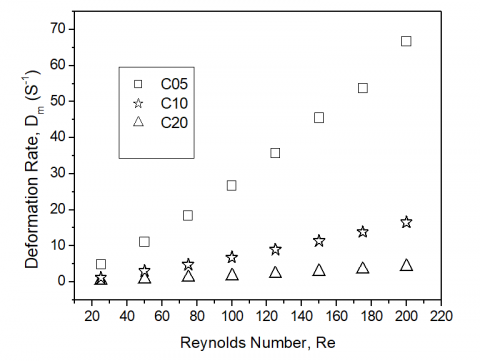Figure 5. Evolutions of the deformation rate with Reynolds number for the three geometries

## figure_6.png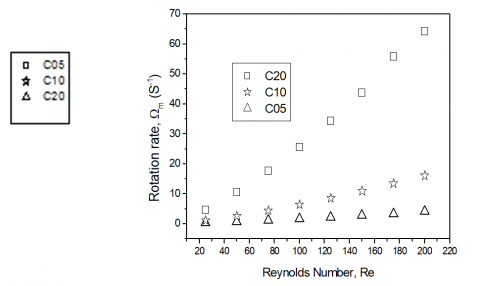Figure 6. Evolutions of the rotation rate with Reynolds number for the three geometries

3.3 Secondary flows

### 3.3.1 Vortex intensity

When the fluid passes through the geometrical perturbation in the considered channel, a secondary flow is created by the presence of a centrifugal force. The secondary flow is more intense for the disturbance having a complex shape. In order to estimate the secondary flow, the vortex intensity was calculated at the exits of the two perturbation zones (plane-4 and plane-8) as defined by :

$\Omega_{\text { average }}=\frac{1}{s} \int\left|\Omega_{Z}\right| d S$(9)

where S is the cross section area and $\Omega_{Z}$  is the vorticity at the flow cross section. Due to the secondary flow effect, the transversal movements of the particles increases and the axial dispersion decreases, which consequently enhanced the heat transfer [23-24]. Figure 7 and 8 display the evolutions of the vortex Intensity $\Omega_{\text { average }}$  with Reynolds number at the exits of the two perturbation areas (plane-4 and plane-8) in the considered geometries. The vortex intensity increases monotonously with the Reynolds number in all the proposed geometries and it increases with the reduction of the geometry scale. An important notice is that the slope of the curve for the geometry C05 is greater than the slopes of the two other curves (C10 and C20). This can be explained by the existence of much great secondary flows in the C05 geometry compared to those exhibited in the other geometries even at low Reynolds numbers. In the geometries C10 and C20, the evolutions are very close to each other.  For a same geometry and at a given Reynolds number, the magnitude of the vortex intensity in the two cross sections (plane-4 and plane-8) is unchangeable which explain that the secondary flow field is uniformly distributed over the entire fluid domain.

## figure_7.pngFigure 7. Variation of the vortex Intensity with Reynolds number for the three geometries at the plane-4 position

## figure_8.pngFigure 8. Variation of the vortex Intensity with Reynolds number for the three geometries at the plane-8 position

3.3.2 Helicity

The helicity, defined as the inner product of velocity and vorticity (Equation 8), characterizes the helical motions. Non zero-helicity declares that the fluid particles move along their axis of rotation and the flow might be expected to be less complex than flows with nonzero Helicity.

$H=\frac{\vec{V} \cdot \vec{\Omega}}{V \Omega}$(10)​

As defined, when the vorticity vector is parallel to the velocity vector the velocity field is nonintegrable and consequently the streamlines are chaotic . Dimensionless helicity is used in the present work to describe the nature of the secondary flows (chaotic or regular), which occur at the exit of each perturbation area (plane-4 and plane-8).  The dimensionless Helicity value is bounded between -1 and +1. For both limit values -1 and +1, the fluid flow is considered fully chaotic. Figures 9 and 10 shows the dimensionless Helicity contours at the plane-4 and plane-8 in the considered geometries C05, C10 and C20, for two Reynolds numbers (100 and 200) respectively. The results shows that, for the same plane and at a given Reynolds number, the dimensionless Helicity contours are identical in all the geometries, which proves that the kinematic flow is unchangeable with the geometry scale variation. According to above, the flow regime in the core region is chaotic for all the presented cases (Figure 9), since the dimensionless Helicity in these zones takes the values of -1 or +1. The direction of the flow rotation in plane-4 is opposite to the flow rotation direction in plane-8. Changing the direction of the curl in the fluid flows provides new opportunities to mix well the fluids.

## figure_9.pngFigure 9. Dimensionless helicity contours at plane-4 for two Reynolds numbers for the three geometries

## figure_10.pngFigure 10. Dimensionless helicity contours at plane-8 for two Reynolds numbers for the three geometries

3.4 Pressure losses

Because the increase of the performances is accompanied by the creation of the pressure drops in all three geometry, it is necessary to estimate this parameter over all Reynolds number range.

The pressure losses of all geometries are characterized by the evolution of the global friction coefficient as function of the Reynolds number defined as:

$C f=\frac{\Delta P}{\frac{1}{2} \rho u_{m}^{2}} \frac{D_{h}}{L}$(11)

where $\rho$  is the fluid density, $\Delta P$  is the pressure difference between inlet and outlet of the geometry, $D_{h}$  is the hydraulic diameter, $u_{m}$  is the mean velocity and L is the curvilinear coordinate. This coefficient presents the ratio between the pressure drop and the kinetic energy.

Figure 11 presents the evolution of the global friction coefficient with Reynolds number in the considered geometries

## figure_11.pngFigure 11.  Evolutions of the mean friction coefficient with Reynolds number Re in the three geometries

The global friction coefficient decreases as function of the Reynolds number in considered geometries. The all curves have identical evolutions. This reflects that there are no significant difference pressure losses between the three geometries. We can see clearly that the evolutions of this parameter with Reynolds number are similar to that obtained in the straight channel .

Because this parameter depends on the Reynolds number, it is preferable to follow the evolution of the product $C f . R e$ , which is the Poiseuille number $P o$ , see figure 12.

## figure_12.pngFigure 12.  Evolutions of the mean friction coefficient with Reynolds number Re in the three geometries

The Poiseuille number increases linearly as a function of the Reynolds number in the three geometries and the curves are perfectly identical and superimposed. We can see that these parameters do not depend to the geometry scale but they depend to the aspect ratio of the geometry. This result presents an important propriety worthy of research.

4. Conclusions

In this paper, numerical study is carried out to examine the influence of the geometry scale on the kinematic behaviour of the fluid flow. Three geometries were considered: C05, C10 and C20 according to their hydraulic diameters equal to 5mm, 10mm and 20mm respectively. The fluid flow behaviour was characterized in terms of the deformation rate, rotation rate, dimensionless helicity contours, the stretching/folding coefficients of the flow vortex and the pressure losses. The dimensionless Helicity contours exhibited a great similarity between the three geometries in terms of the secondary flow structure, which reflects the similarity between the geometry scale and the cartography of the flow structure.  In addition, these contours showed that, in the central regions, the vorticity and the velocity vectors are parallels; these outcomes indicate the fully chaotic regime of the fluid flow in these zones. Besides, the C05 geometry revealed a great deformation and rotation rates compared to the others (C10 and C20). As known, the enhancement of these parameters (deformation and rotation rates) in the fluid flow maximizes the mixing level of the fluids. Furthermore, the performed study explored the effect of the geometry scale on the stretching/compression coefficients of the vortexes and it was found that C05 geometry exhibited the higher values.

The increase of the performances of such geometries is penalised, generally, by the increase of the pressure drops in the flow. In this study, the calculation of the pressure losses confirms that all geometries have an identical friction coefficient where this later depends only to the aspect ratio of the geometry.

This contributes enormously to improve the fluid flow performance in terms of heat transfer and fluid mixing of such geometry without energy expenditure. As a conclusion, the diminution of the geometry scale presents a key to boost the local physical process of the flow in the considered geometry in terms of deformation and rotation rates, stretching and compression coefficients, even if the flow regime is laminar.

Nomenclature
 Cf Global friction coefficient D Deformation rate, s-1 Dm Mean deformation rate, s-1 Dh Hydraulic diameter, m $\overline{\overline{D}}$ Deformation tensor H Dimensionless helicity Po Poiseuille number Re Reynolds number S Cross section m2 V Velocity, m.s-1 u, v, w Velocity components in the x, y and z directions, m.s-1 Um Mean velocity, m.s-1 Greek symbols $\alpha$ Stretching/compression coefficient $\Delta \mathrm{P}$ pressure Difference, Pa $v$ Kinematic viscosity, m2.s-1 $\rho$ Density of fluid, kg.m-3 $\Omega$ Rotation rate, s-1 $\Omega_{m}$ Mean rotation rate, s-1 $\Omega_{Z}$ Rotation rate in the cross section,s-1 $\mho$ Volume of fluide, m3
References

 T. T. Naas, Y. Lasbet, A. Aidaoui and K. Loubar, “Characterization of Pressure Drops and Heat Transfer of Non-Newtonian Power-Law Fluid Flow Flowing in Chaotic Geometry,” International Journal of Heat and Technology, vol. 34, no, 3, pp. 251–260, 2016. DOI: 10.18280/ijht.340214.

 H. Aref, “Stirring by chaotic advection,” J Fluid    Mech, vol. 143, pp. 1–21, 1984. DOI: 10.1017/S0022112084001233.

 A. Tohidi, S. M. Hosseinalipour, M. Shokrpour, A.S.Mujumdar, “Heat transfer enhancement utilizing chaotic advection in coiled tube heat exchangers,” Appl Therm Eng, vol. 76, pp. 185–195, 2015. DOI: 10.1016/j.applthermaleng.2014.10.073.

 C. Castelain, Y. Lasbet, B. Auvity and H. Peerhossaini, “Experimental study of the thermal performance of chaotic geometries for their use in PEM fuel cells,” International Journal of Thermal Sciences, vol. 101, pp. 114–191, 2016.  DOI: 10.1016/j.jpowsour.2005.08.030.

 D. Kaliakatsos, M. Cucumo, V. Ferraro, M. Mele, A. Galloro and F. Accorinti, “CFD Analysis of a Pipe Equipped with Twisted Tape,” International Journal of Heat and Technology, vol. 34, no. 2, June 2016, pp. 172-180, 2016. DOI: 10.18280/ijht.340203.

 A. A. Gourjii, “Intensive and weak mixing in the chaotic region of velocity field,” in Tubes, Sheets and Singularities in Fluid Dynamics, vol. 71, E. M. Sparrow, Y. I. Cho, J. P. Abraham and J. M. Gorman, eds., Burlington, USA: Academic Press, 2002, pp. 87-92. DOI: 10.1007/0-306-48420-X.

 F. E. Marble, “Growth of a diffusion flame in the field of a vortex,” in Recent Advances in the Aerospace Sciences, C. Casci, B. Corrado, eds., Burlington, USA: Springer US, 1985, pp. 395-413. DOI: 10.1007/978-1-4684-4298-4_19.

 V. Toussaint, C. Philippe and R. Florence, “A numerical Eulerian approach to mixing by chaotic advection,” Phys Fluids., vol. 7, pp. 2587-2600, 1995. DOI: 10.1063/1.868707.

 F. Raynal and J. Gence, “Energy saving in chaotic laminar mixing,” Int J Heat and Mass Tranf, vol. 40, no. 14, pp.  3267-3273, 1997. DOI: 10.1016/S0017-9310(96)00383-3.

 S. Jones, O. Thomas and H. Aref, “Chaotic advection by laminar flow in a twisted pipe,” J Fluid Mechanics., vol. 209, pp. 335–357, 1989. DOI: 10.1017/S0022112089003137.

 C. Habchi, T. Lemenand, D. Della Valle and H. Peerhossaini, “Liquid-liquid dispersion in a chaotic advection flow,” In J Multiphase Flow., vol. 35, pp. 485–497, 2009. DOI: 10.1016/j.ijmultiphaseflow.2009.02.019.

 C. Castelain, A. Mokrani, Y. Le Guer and H. Peerhossaini, “Experimentaly study of chaotic advection regime in a twisted duct flow,” European Journal of Mechanics B Fluids., vol. 20, pp. 205–232, 2001. DOI: 10.1016/S0997-7546(00)01116-X.

 C. Habchi, J. L. Harion, S. Russeil, D. Bougeard, F. Hachem and A. Elmarakbi, “Chaotic mixing by longitudinal vorticity,” Chemical Engineering Science., vol. 104, pp. 439–450, 2013. DOI: 10.1016/j.ces.2013.09.032.

 Y. Lasbet C. Castelain, B. Auvity and H. Peerhossaini, “A chaotic heat-exchanger for PEMFC cooling applications,” Journal of Power Sources., vol. 156, pp. 114–118, 2006. DOI: 10.1016/j.jpowsour.2005.08.030.

 Y. Lasbet C. Castelain, B. Auvity and H. Peerhossaini, “Thermal and hydrodynamic performances of chaotic mini-channel: Application to the fuel cell cooling,” Heat Transfer Engineering, vol. 28, no. 8-9, pp. 795–803, 2007. DOI: 10.1080/01457630701328908.

 R. H. Liu, M. A. Stremler, K. V. Sharp, M. G. Olsen, J. G. Santiago, R. J. Adrian, H. Aref and D. J. Beeb, “Passive mixing in a three-dimensional serpentine microchannel,” Journal of Microelectromechanical Systems., vol. 9, pp. 190–197, 2000.  DOI: 10.1109/84.846699.

 D. J. Beebe, R. J. Adrian, M. G. Olsen, M. A. Stremler, H. Aref and B. Jo, “Passive mixing in micro-channels: fabrication and flow experiments,” Mécanique et Industries., vol. 2, pp. 343–348, 2001.

 R. K. Shah and A. London, “Rectangular ducts” in A Laminar Flow Forced Convection in Ducts, T. F. Irvine, J. P. Hartnett, eds., New York, USA: Academic Press, 1978, pp. 196-219.

 K. El Omari, Y. Le Guer, “Alternate rotating walls for thermal chaotic mixing,” International Journal of Heat and Mass Transfer., vol. 53, no. 1-3, pp. 123–134, 2010. DOI:  10.1016/j.ijheatmasstransfer.2009.09.046.

 T. Lemenand, C. Habchi, D. Della Valle, J. Bellettre,H. Peerhossaini, “Mass transfer and emulsification by chaotic advection,” International Journal of Heat and Mass Transfer., vol. 71, pp. 228–235, 2014. DOI: 10.1016/j.ijheatmasstransfer.2013.12.015.

 B. Galanti, J. D. Gibbonyzk and M. Heritagez, “Vorticity alignment results for the three-dimensional Euler and Navier–Stokes equations,”Nonlinearity, vol. 10, pp. 1675–1694, 1997. DOI: 10.1088/0951-7715/10/6/013.

 D. V. Khakhar and J. M. Ottino, “Deformation and breakup of slender drops in linear flows,” J Fluid Mech., vol. 166, pp. 265–285, 1986. DOI:  10.1017/S0022112086000149.

 L. M. Chang, L. B. Wang, K. W. Song, D. L. Sun and J. F. Fan, “Numerical study of the relationship between heat transfer enhancement and absolute vorticity flux a long main flow direction in a channel formed by a flat tube bank fin with vortex generators,” International Journal of Heat and Mass Transfer., vol, 52, pp. 1794–1801, 2009. DOI:  10.1016/j.ijheatmasstransfer.2008.09.029.

 W. L. Hu, K. W. Song, Y. Guan, L. M. Chang, S. Liu and L. B. Wang, “Secondary flow intensity determines Nusselt number on the fin surfaces of circle tube bank fin heat exchanger,” International Journal of Heat and Mass Transfer., vol. 62, pp. 620–631, 2013. DOI: 10.1016/j.ijheatmasstransfer.2013.03.011.

 V. Arnold, “Sur la topologie des écoulements stationnaires des fluides parfaits,” in Collected works, A. B. Givental, B. A. Khesin, A. N. Varchenko, V. A. Vassiliev, O. Ya. Viro, eds., Heidelberg, Germany: Springer Berlin, 2013, pp. 15-18.  DOI: 10.1007/978-3-642-31031-7.